# NCERT Solutions for Class 7 Maths Chapter 12 – Algebraic Expressions

Class 7 Maths NCERT Solutions Chapter 12 – Algebraic Expressions comprises of the 4 Exercises

This Chapter contains the Exercises relating to the following topics , which are discussed in Chapter 12 – Algebraic Expressions Class 7 NCERT book  : –

• 12.1 INTRODUCTION
• 12.2 HOW ARE EXPRESSIONS FORMED?
• 12.3 TERMS OF AN EXPRESSION
• 12.4 LIKE AND UNLIKE TERMS
• 12.5 MONOMIALS, BINOMIALS, TRINOMIALS AND POLYNOMIALS
• 12.6 ADDITION AND SUBTRACTION OF ALGEBRAIC EXPRESSIONS
• 12.7 FINDING THE VALUE OF AN EXPRESSION
• 12.8 USING ALGEBRAIC EXPRESSIONS – FORMULAS AND RULES

### NCERT Solutions for Class 7 Maths Chapter 12 Exercise 12.1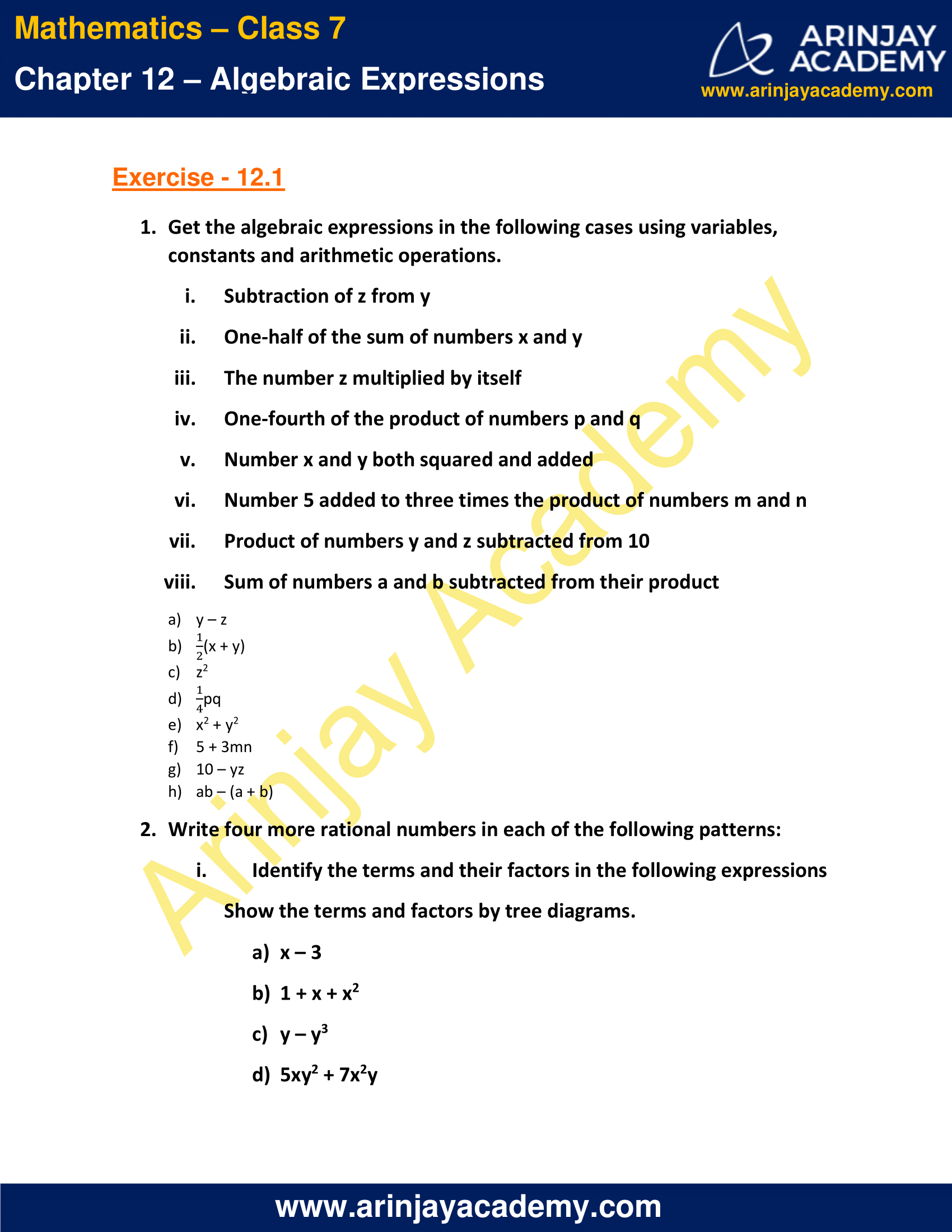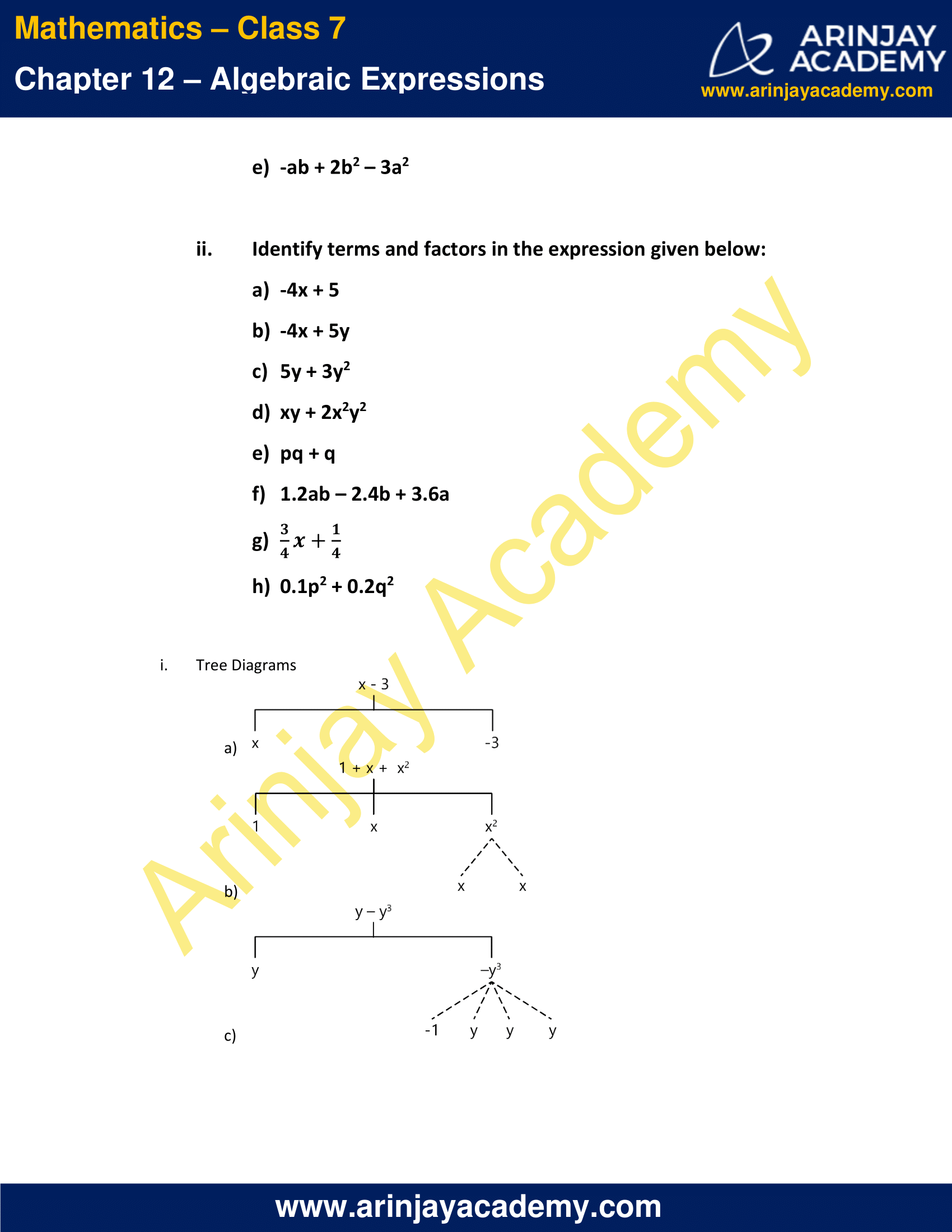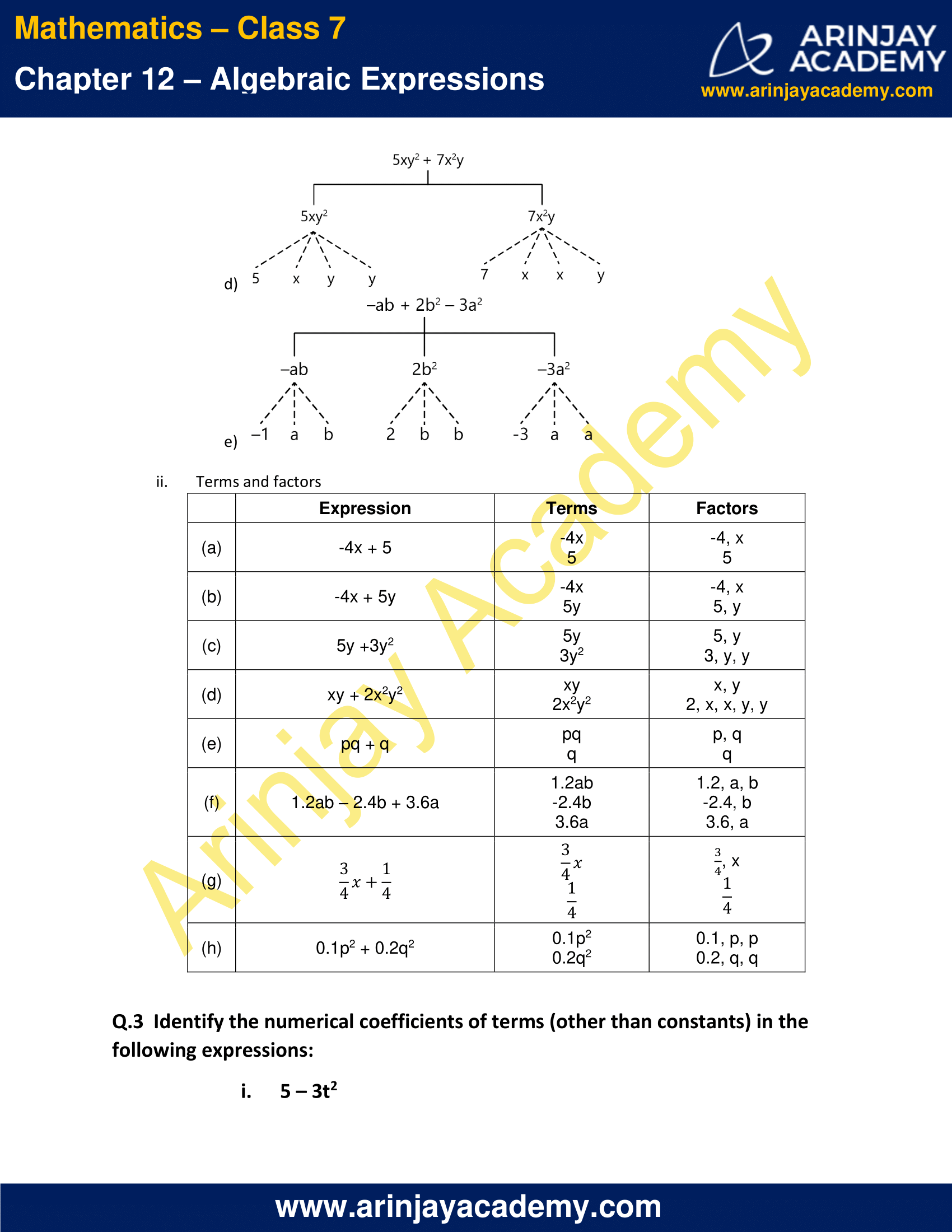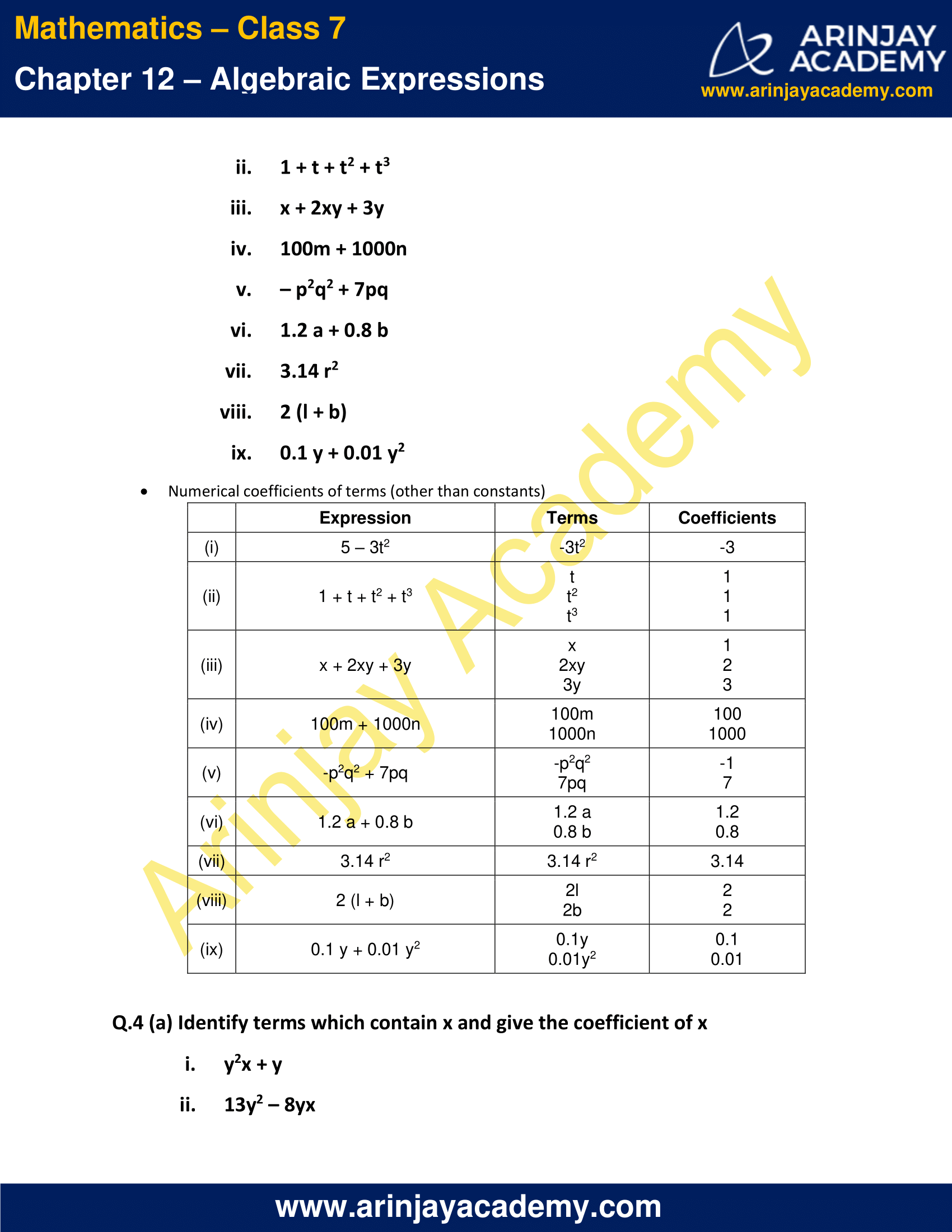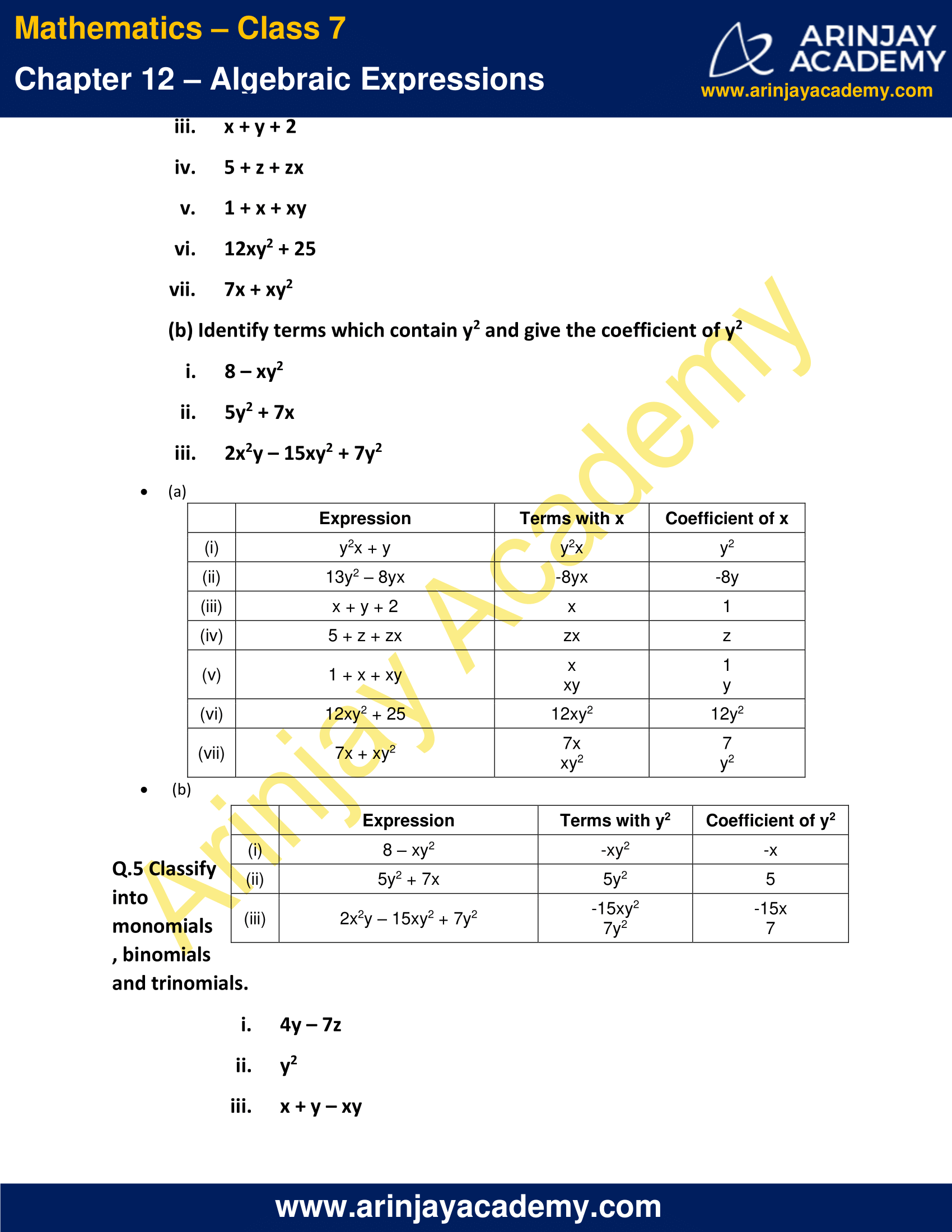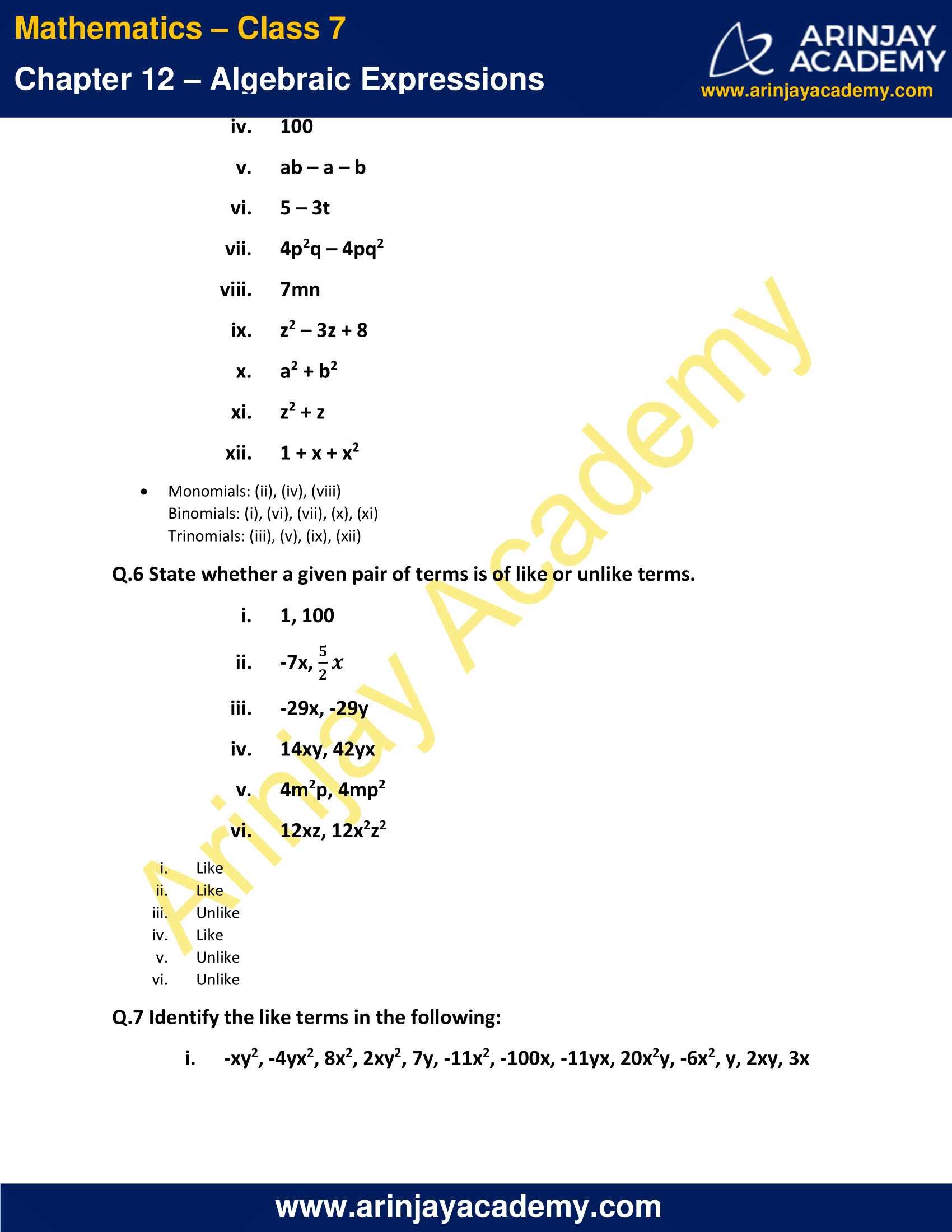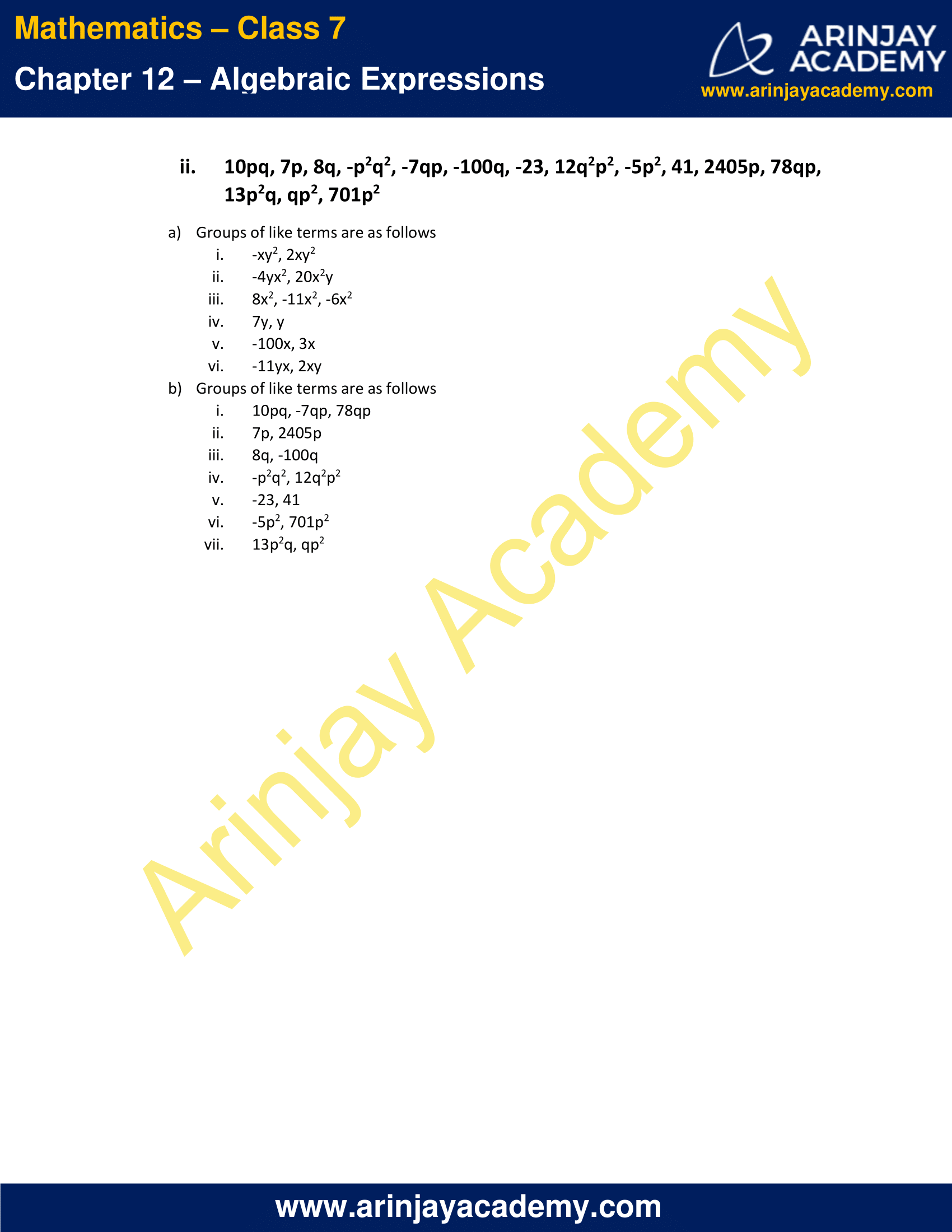### NCERT Solutions for Class 7 Maths Chapter 12 Exercise 12.2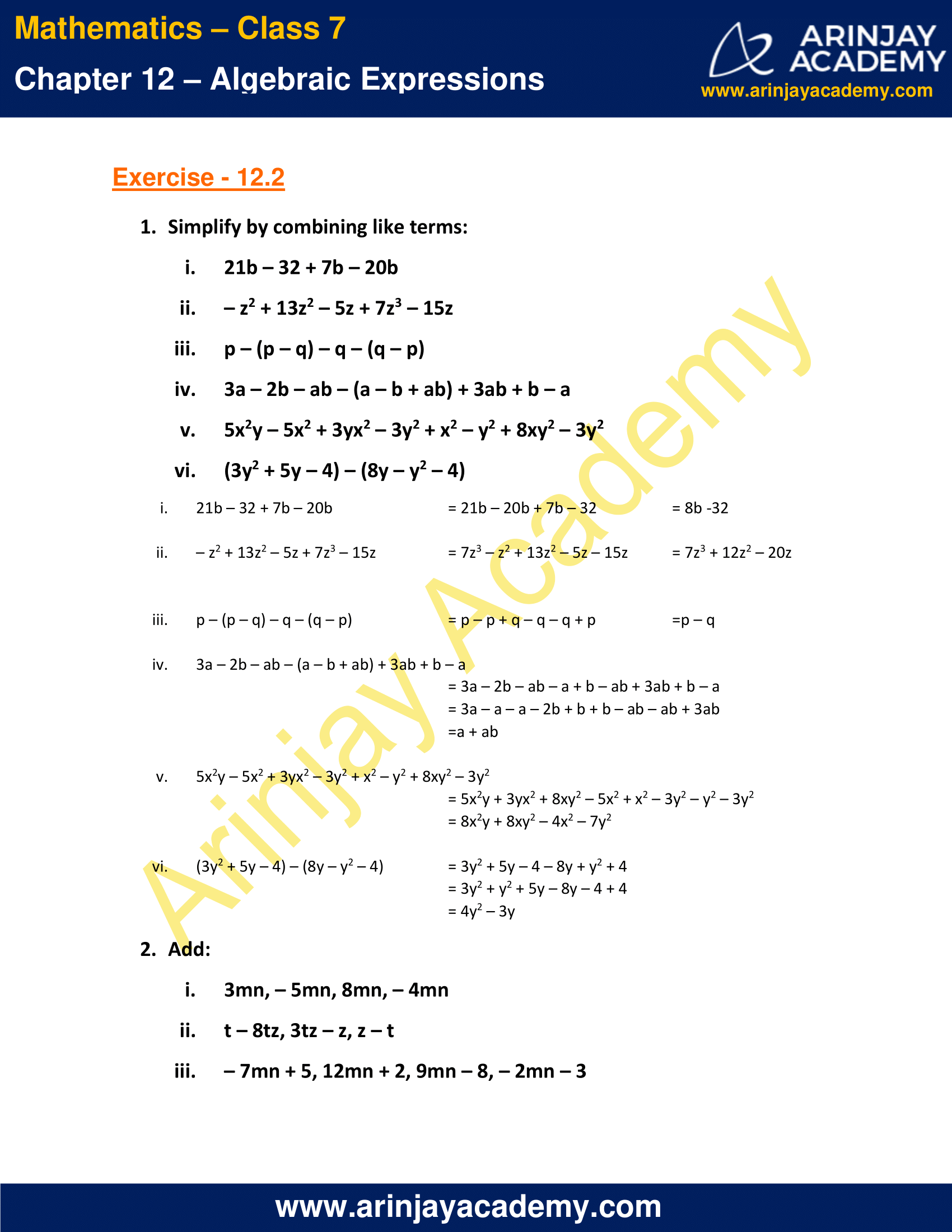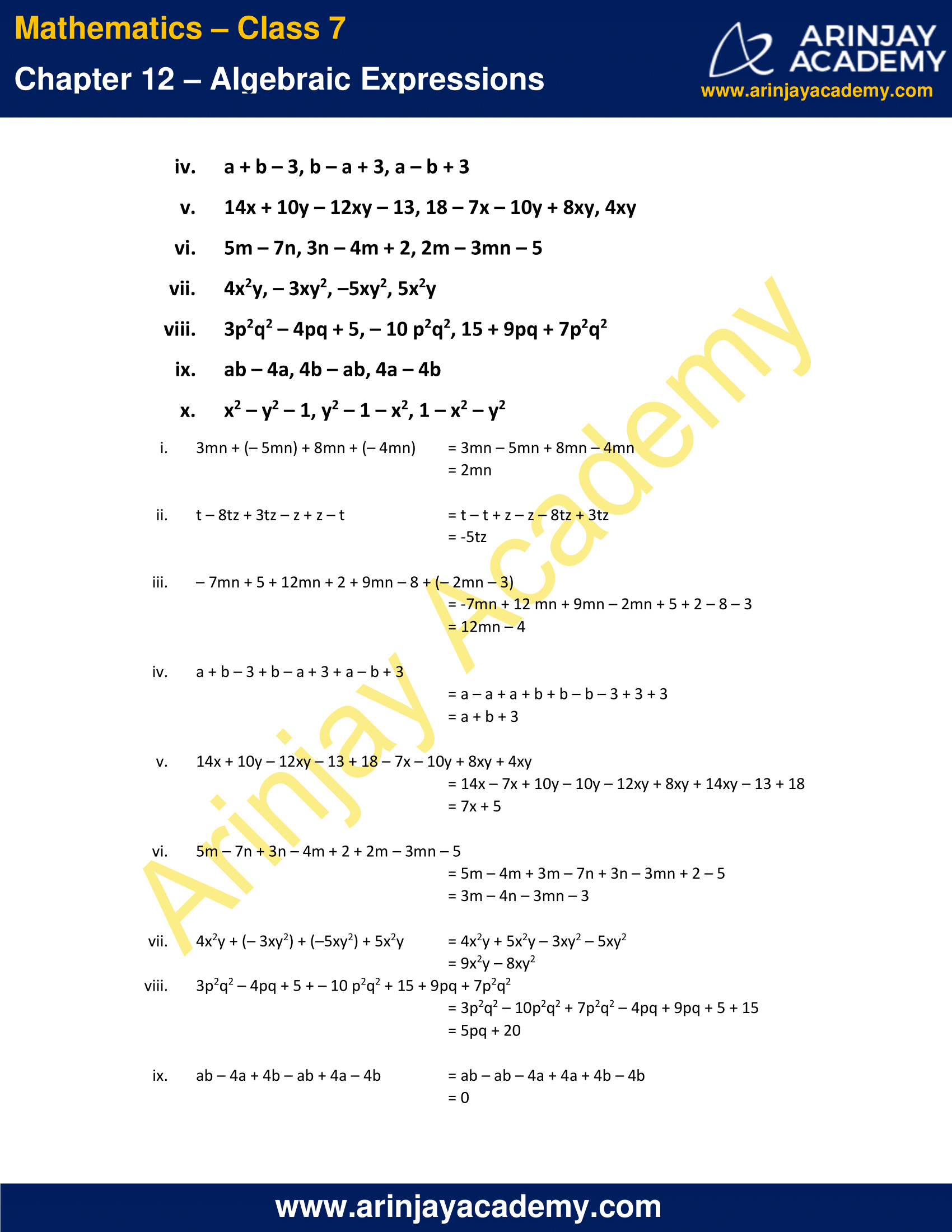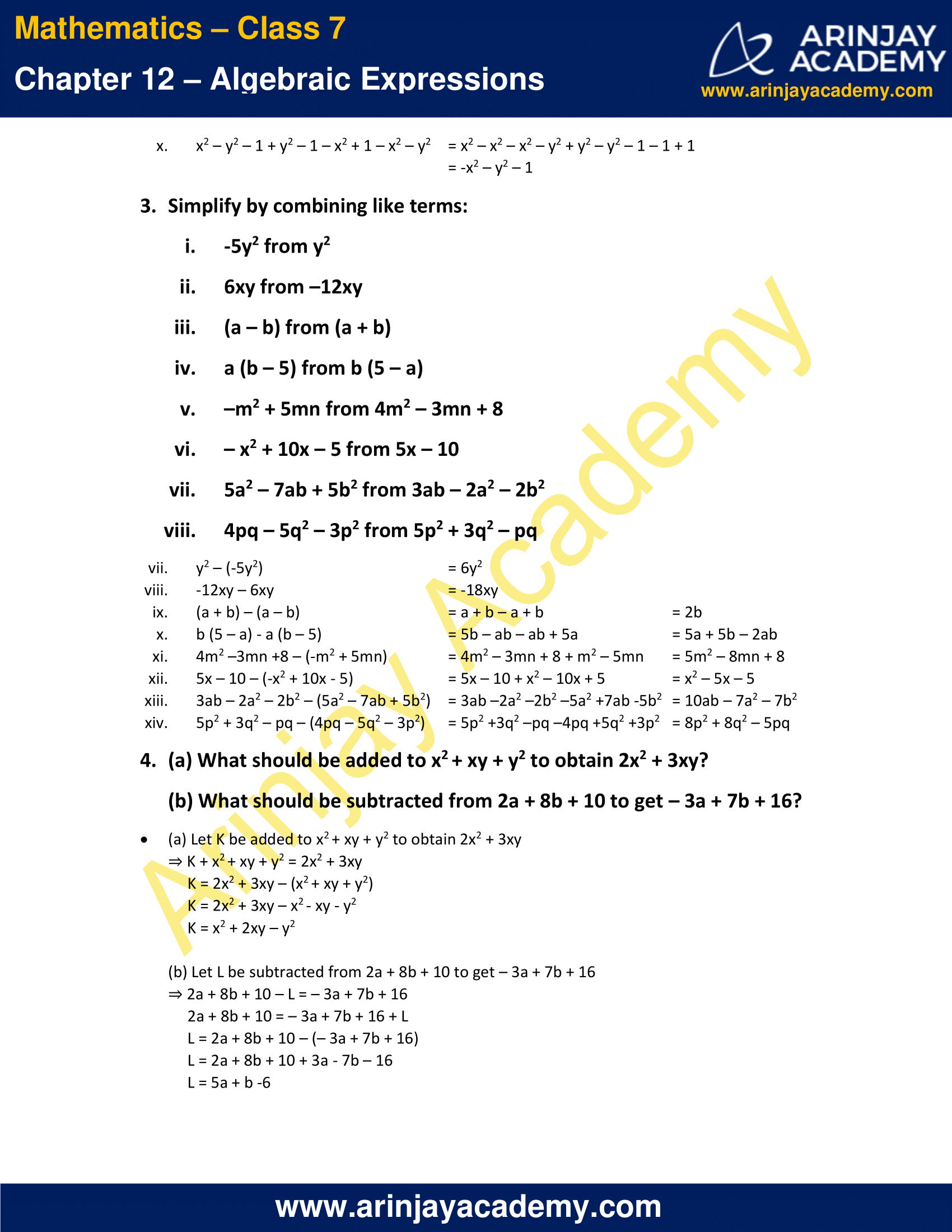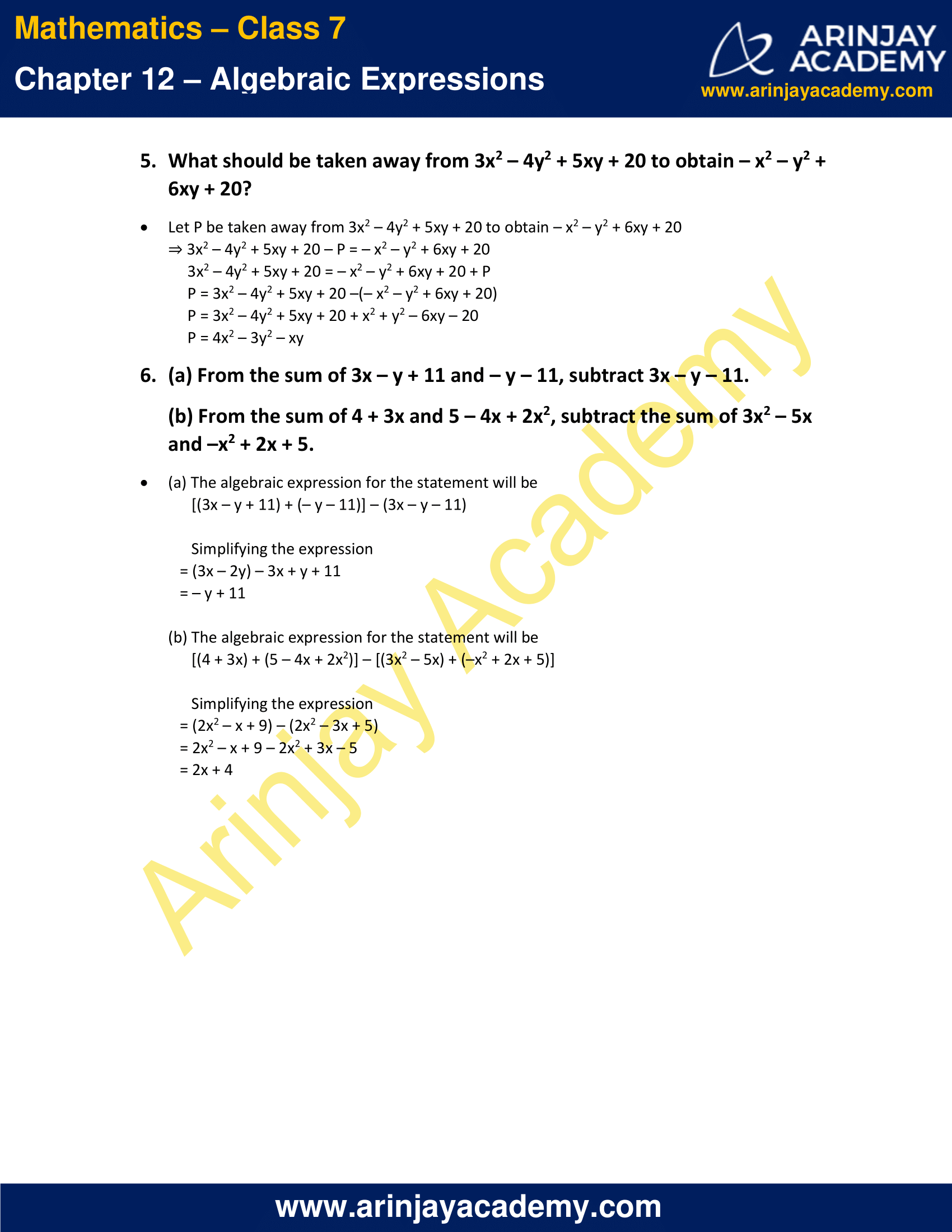### NCERT Solutions for Class 7 Maths Chapter 12 Exercise 12.3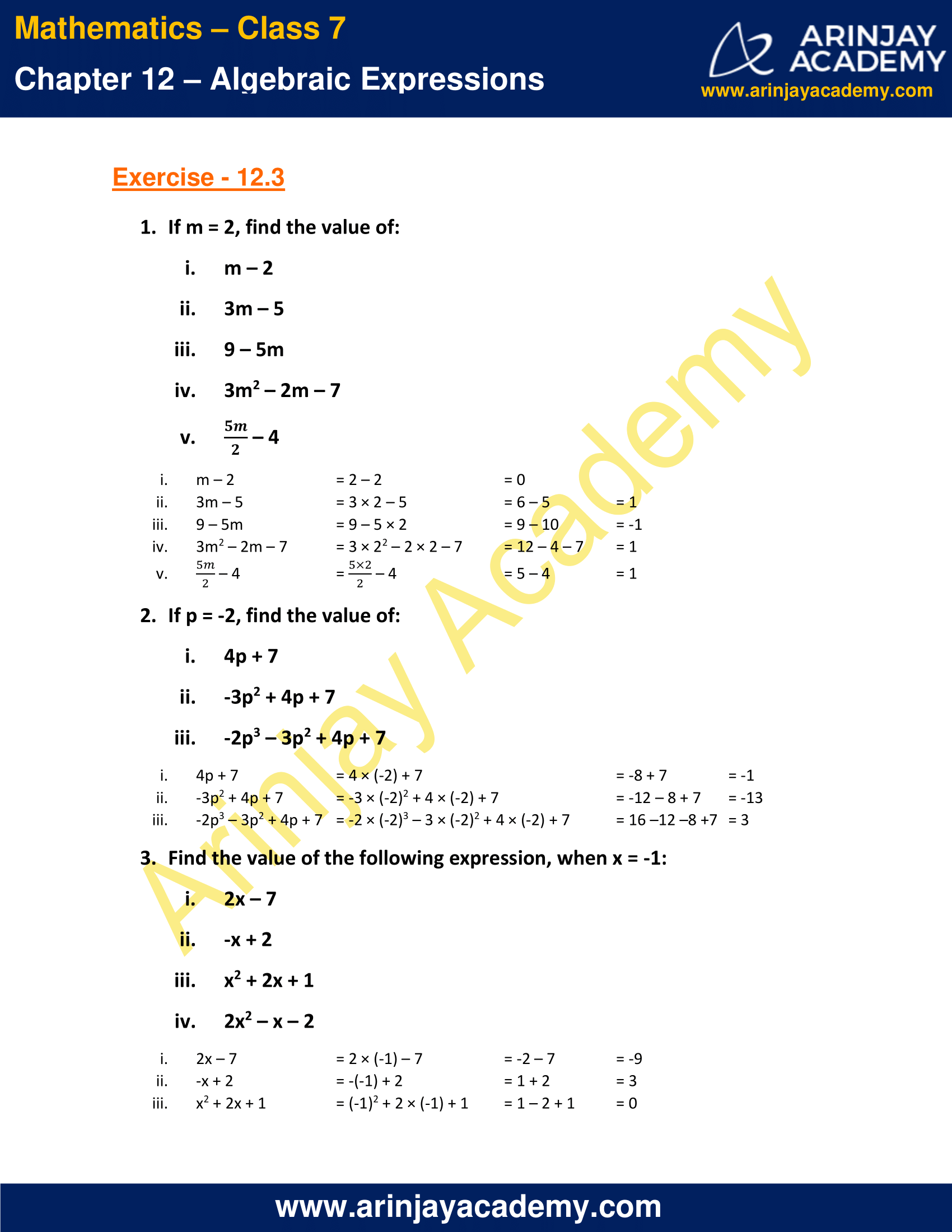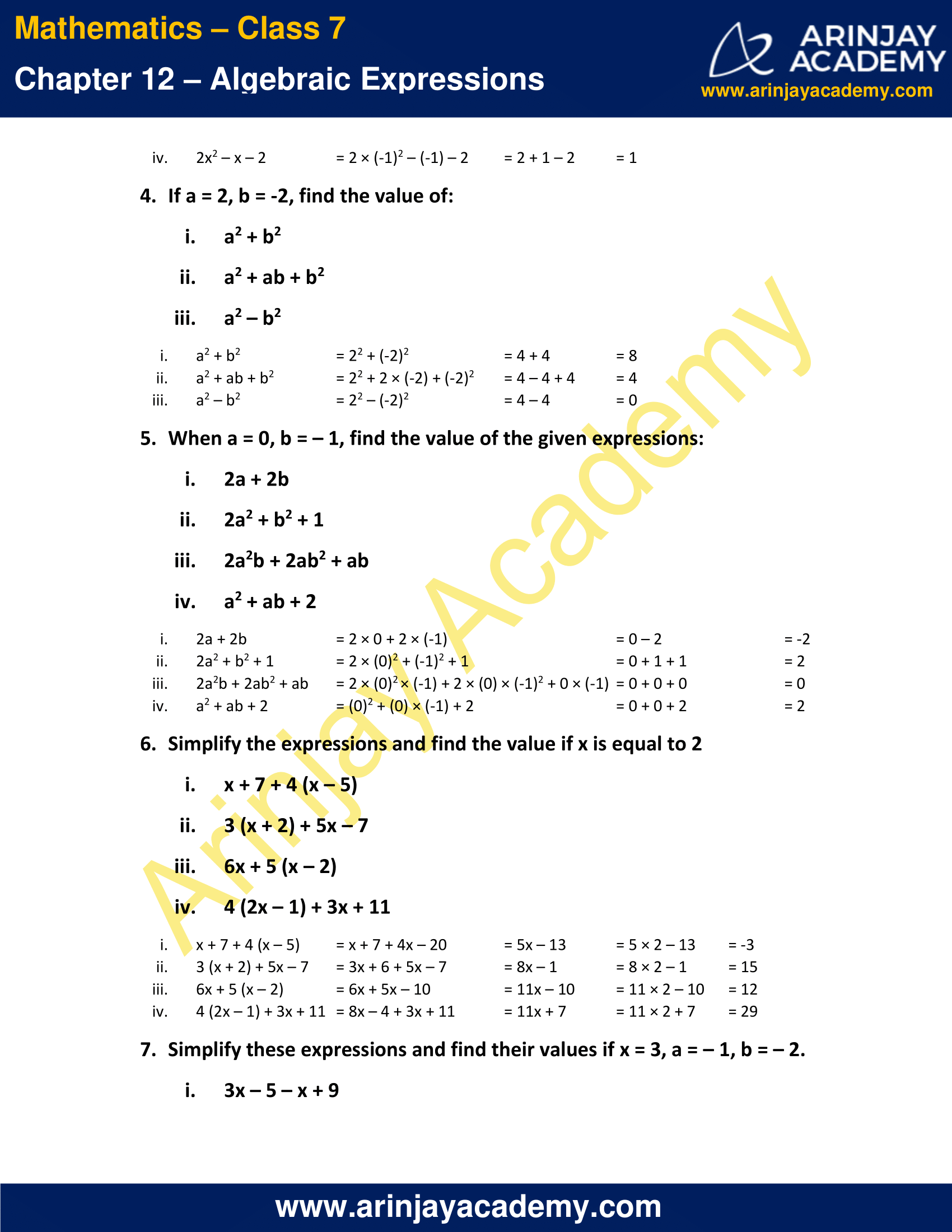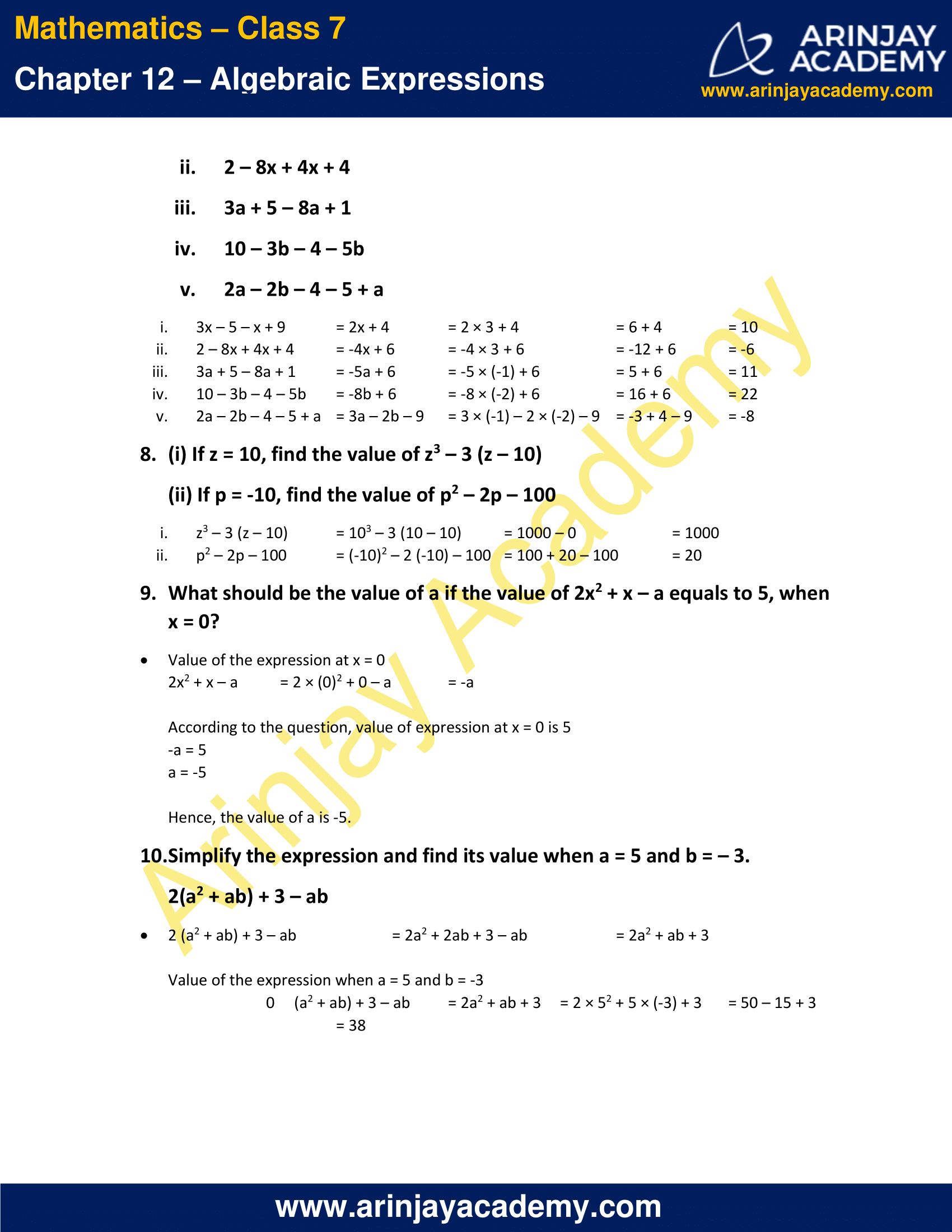### NCERT Solutions for Class 7 Maths Chapter 12 Exercise 12.4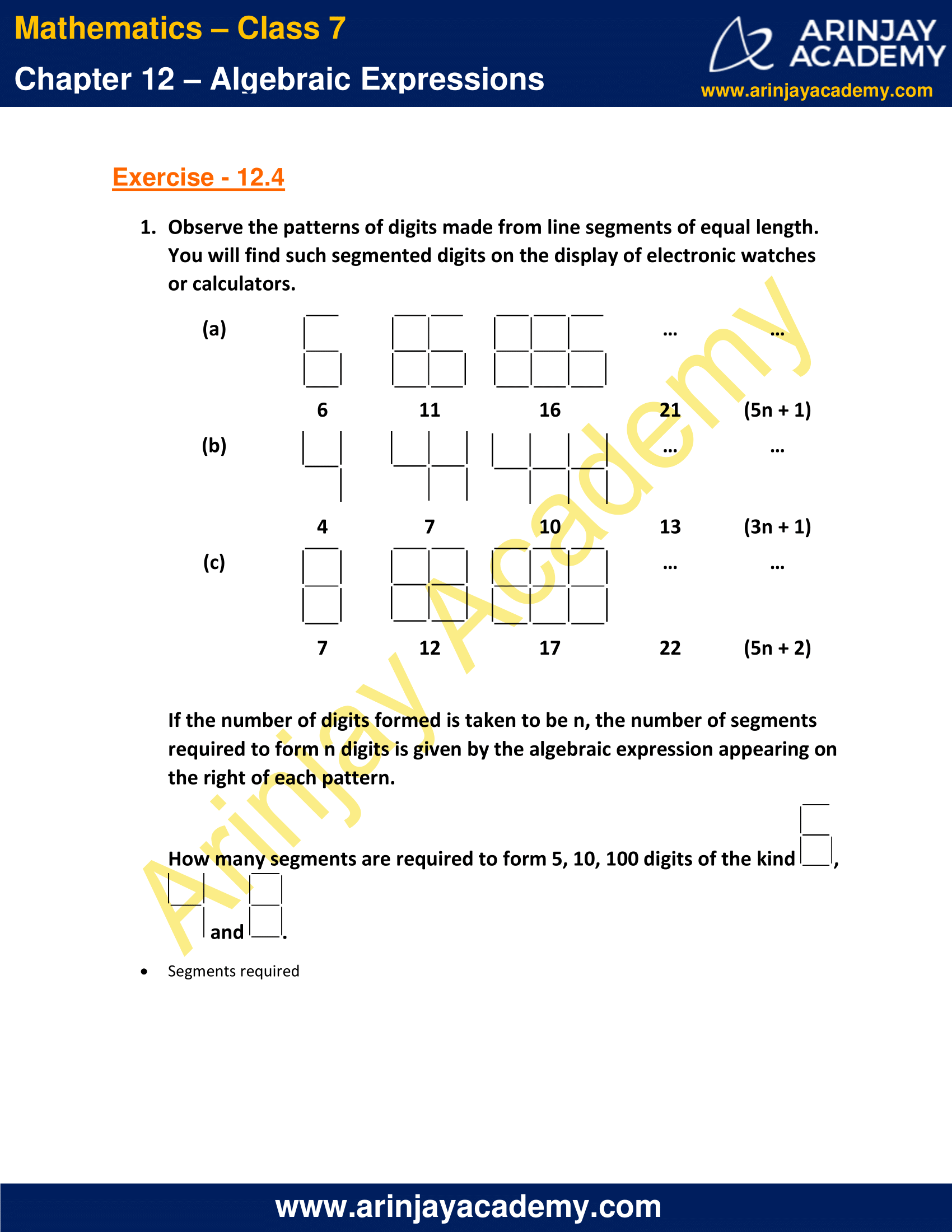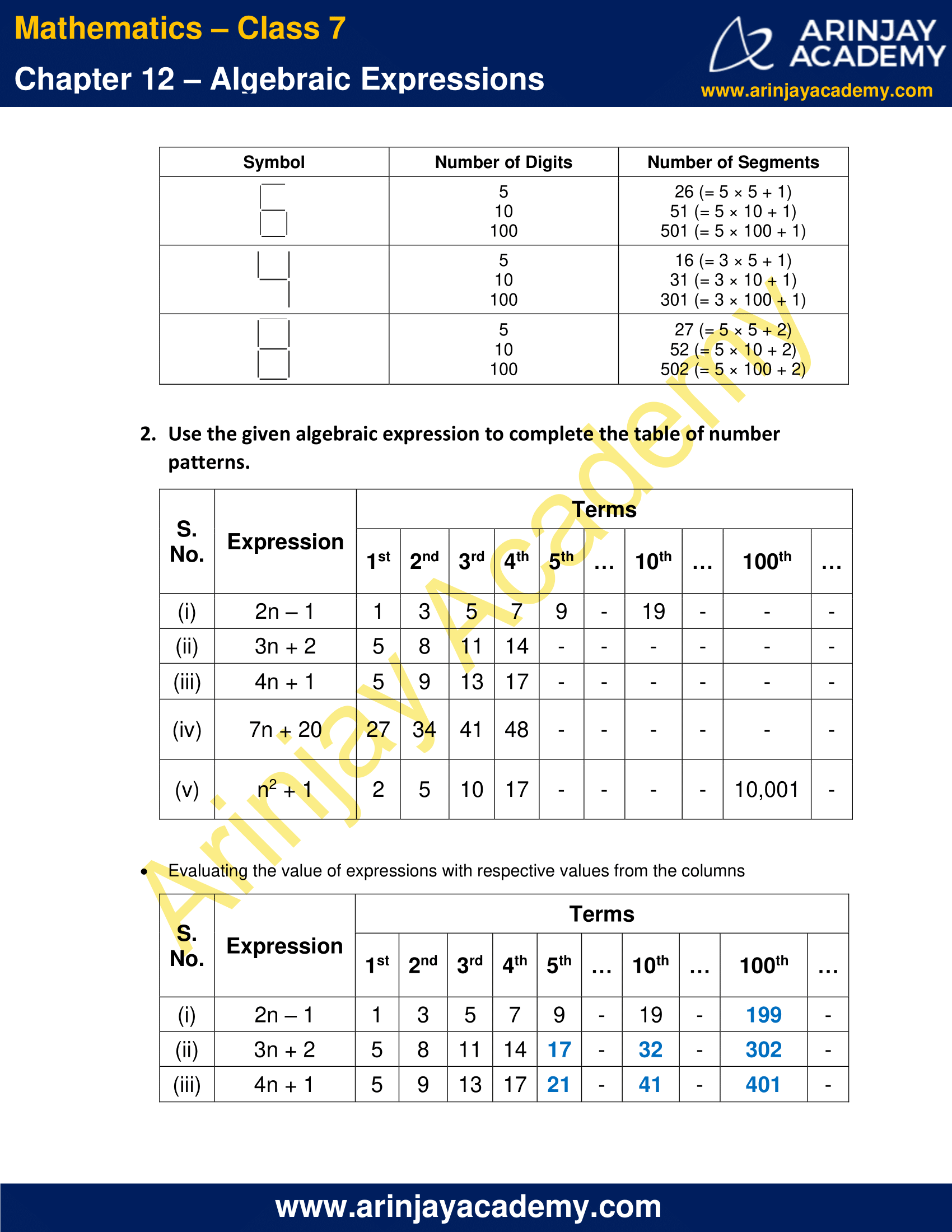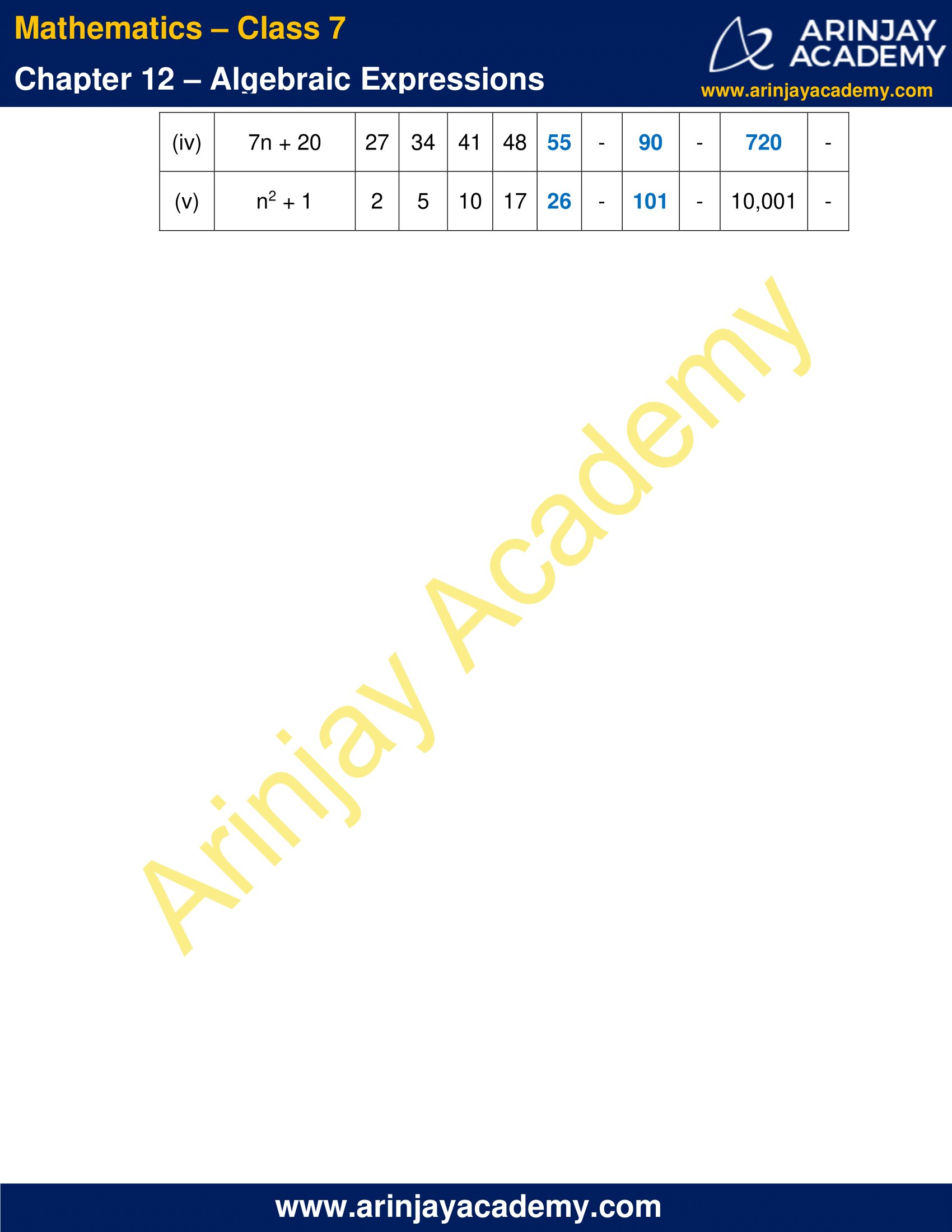### NCERT Solutions for Class 7 Maths Chapter 12 Exercise 12.1

1. Get the algebraic expressions in the following cases using variables, constants and arithmetic operations.

a) Subtraction of z from y
b) One-half of the sum of numbers x and y
c) The number z multiplied by itself
d) One-fourth of the product of numbers p and q
e) Number x and y both squared and added
f) Number 5 added to three times the product of numbers m and n
g) Product of numbers y and z subtracted from 10
h) Sum of numbers a and b subtracted from their product

Solution:

a) y – z
b) 1/2(x + y)
c) z2
d) (1/4)pq
e) x2 + y2
f) 5 + 3mn
g) 10 – yz
h) ab – (a + b)

2. Write four more rational numbers in each of the following patterns:

i. Identify the terms and their factors in the following expressions
Show the terms and factors by tree diagrams.
a) x – 3
b) 1 + x + x2
c) y – y3
d) 5xy2 + 7x2y
e) -ab + 2b2 – 3a2

ii. Identify terms and factors in the expression given below:

a) -4x + 5
b) -4x + 5y
c) 5y + 3y2
d) xy + 2x2y2
e) pq + q
f) 1.2ab – 2.4b + 3.6a
g) (3/4)x + (1/4)
h) 1p2 + 0.2q2

Solution:

i. Tree Diagrams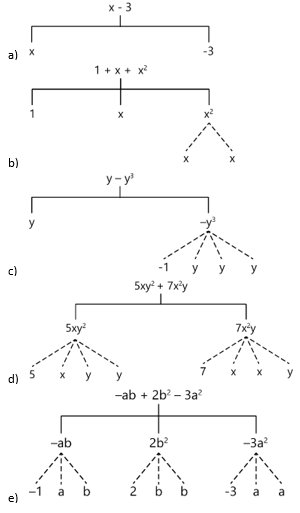ii. Terms and factors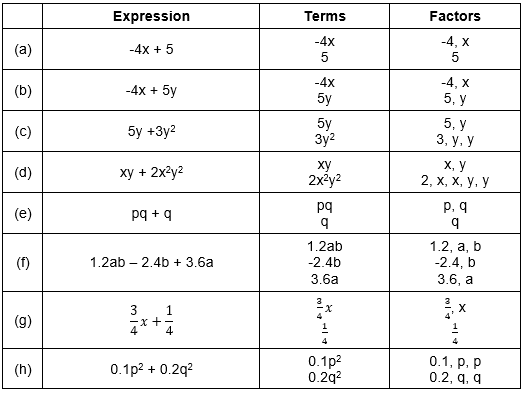Q.3  Identify the numerical coefficients of terms (other than constants) in the following expressions:

i. 5 – 3t2
ii. 1 + t + t2 + t3
iii. x + 2xy + 3y

iv. 100m + 1000n
v. – p2q2 + 7pq
vi. 1.2 a + 0.8 b
vii. 3.14 r2
viii. 2 (l + b)

ix. 0.1 y + 0.01 y2

Solution:

Numerical coefficients of terms (other than constants)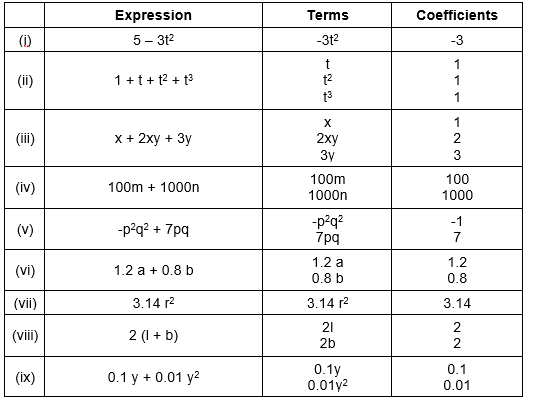Q.4 (a) Identify terms which contain x and give the coefficient of x

i. y2x + y
ii. 13y2 – 8yx
iii. x + y + 2
iv. 5 + z + zx
v. 1 + x + xy
vi. 12xy2 + 25
vii. 7x + xy2

(b) Identify terms which contain y2 and give the coefficient of y2

i. 8 – xy2
ii. 5y2 + 7x

iii. 2x2y – 15xy2 + 7y2

Solution:

a)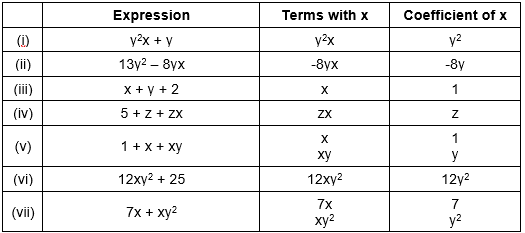b)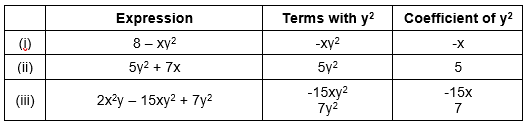Q.5 Classify into monomials, binomials and trinomials.

i. 4y – 7z
ii. y2
iii. x + y – xy

iv. 100
v. ab – a – b
vi. 5 – 3t
vii. 4p2q – 4pq2
viii. 7mn

ix. z2 – 3z + 8
x. a2 + b2
xi. z2 + z

xii. 1 + x + x2

Solution:

Monomials: (ii), (iv), (viii)
Binomials: (i), (vi), (vii), (x), (xi)
Trinomials: (iii), (v), (ix), (xii)

Q.6 State whether a given pair of terms is of like or unlike terms.

i. 1, 100
ii. -7x, (5/2)x
iii. -29x, -29y
iv. 14xy, 42yx
v. 4m2p, 4mp2
vi. 12xz, 12x2z2

i. Like
ii. Like
iii. Unlike
iv. Like
v. Unlike
vi. Unlike

Q.7 Identify the like terms in the following:

a) -xy2, -4yx2, 8x2, 2xy2, 7y, -11x2, -100x, -11yx, 20x2y, -6x2, y, 2xy, 3x
b) 10pq, 7p, 8q, -p2q2, -7qp, -100q, -23, 12q2p2, -5p2, 41, 2405p, 78qp, 13p2q, qp2, 701p2

a) Groups of like terms are as follows

i. -xy2, 2xy2
ii. -4yx2, 20x2y
iii. 8x2, -11x2, -6x2
iv. 7y, y
v. -100x, 3x
vi. -11yx, 2xy

b) Groups of like terms are as follows

i. 10pq, -7qp, 78qp
ii. 7p, 2405p
iii. 8q, -100q
iv. -p2q2, 12q2p2
v. -23, 41
vi. -5p2, 701p2
vii. 13p2q, qp2

### NCERT Solutions for Class 7 Maths Chapter 12 Exercise 12.2

1. Simplify by combining like terms:

i. 21b – 32 + 7b – 20b
ii. – z2 + 13z2 – 5z + 7z3 – 15z
iii. p – (p – q) – q – (q – p)
iv. 3a – 2b – ab – (a – b + ab) + 3ab + b – a
v. 5x2y – 5x2 + 3yx2 – 3y2 + x2 – y2 + 8xy2 – 3y2
vi. (3y2 + 5y – 4) – (8y – y2 – 4)

i. 21b – 32 + 7b – 20b
= 21b – 20b + 7b – 32
= 8b -32

ii. – z2 + 13z2 – 5z + 7z3 – 15z
= 7z3 – z2 + 13z2 – 5z – 15z
= 7z3 + 12z2 – 20z

iii. p – (p – q) – q – (q – p)
= p – p + q – q – q + p
=p – q

iv. 3a – 2b – ab – (a – b + ab) + 3ab + b – a
= 3a – 2b – ab – a + b – ab + 3ab + b – a
= 3a – a – a – 2b + b + b – ab – ab + 3ab
=a + ab

v. 5x2y – 5x2 + 3yx2 – 3y2 + x2 – y2 + 8xy2 – 3y2
= 5x2y + 3yx2 + 8xy2 – 5x2 + x2 – 3y2 – y2 – 3y2
= 8x2y + 8xy2 – 4x2 – 7y2

vi. (3y2 + 5y – 4) – (8y – y2 – 4)
= 3y2 + 5y – 4 – 8y + y2 + 4
= 3y2 + y2 + 5y – 8y – 4 + 4
= 4y2 – 3y

i. 3mn, – 5mn, 8mn, – 4mn
ii. t – 8tz, 3tz – z, z – t
iii. – 7mn + 5, 12mn + 2, 9mn – 8, – 2mn – 3
iv. a + b – 3, b – a + 3, a – b + 3
v. 14x + 10y – 12xy – 13, 18 – 7x – 10y + 8xy, 4xy
vi. 5m – 7n, 3n – 4m + 2, 2m – 3mn – 5
vii. 4x2y, – 3xy2, –5xy2, 5x2y
viii. 3p2q2 – 4pq + 5, – 10 p2q2, 15 + 9pq + 7p2q2
ix. ab – 4a, 4b – ab, 4a – 4b
x. x2 – y2 – 1, y2 – 1 – x2, 1 – x2 – y2

i. 3mn + (– 5mn) + 8mn + (– 4mn)
= 3mn – 5mn + 8mn – 4mn
= 2mn

ii. t – 8tz + 3tz – z + z – t
= t – t + z – z – 8tz + 3tz
= -5tz

iii. – 7mn + 5 + 12mn + 2 + 9mn – 8 + (– 2mn – 3)
= -7mn + 12 mn + 9mn – 2mn + 5 + 2 – 8 – 3
= 12mn – 4

iv. a + b – 3 + b – a + 3 + a – b + 3
= a – a + a + b + b – b – 3 + 3 + 3
= a + b + 3

v. 14x + 10y – 12xy – 13 + 18 – 7x – 10y + 8xy + 4xy
= 14x – 7x + 10y – 10y – 12xy + 8xy + 14xy – 13 + 18
= 7x + 5

vi. 5m – 7n + 3n – 4m + 2 + 2m – 3mn – 5
= 5m – 4m + 3m – 7n + 3n – 3mn + 2 – 5
= 3m – 4n – 3mn – 3

vii. 4x2y + (– 3xy2) + (–5xy2) + 5x2y
= 4x2y + 5x2y – 3xy2 – 5xy2
= 9x2y – 8xy2

viii. 3p2q2 – 4pq + 5 + – 10 p2q2 + 15 + 9pq + 7p2q2
= 3p2q2 – 10p2q2 + 7p2q2 – 4pq + 9pq + 5 + 15
= 5pq + 20

ix. ab – 4a + 4b – ab + 4a – 4b
= ab – ab – 4a + 4a + 4b – 4b
= 0

x. x2 – y2 – 1 + y2 – 1 – x2 + 1 – x2 – y2
= x2 – x2 – x2 – y2 + y2 – y2 – 1 – 1 + 1
= -x2 – y2 – 1

3. Simplify by combining like terms:

i. -5y2 from y2
ii. 6xy from –12xy
iii. (a – b) from (a + b)
iv. a (b – 5) from b (5 – a)
v. –m2 + 5mn from 4m2 – 3mn + 8
vi. – x2 + 10x – 5 from 5x – 10
vii. 5a2 – 7ab + 5b2 from 3ab – 2a2 – 2b2
viii. 4pq – 5q2 – 3p2 from 5p2 + 3q2 – pq

i. y2 – (-5y2)
= 6y2

ii. -12xy – 6xy
= -18xy

iii. (a + b) – (a – b)
= a + b – a + b
= 2b

iv. b (5 – a) – a (b – 5)
= 5b – ab – ab + 5a
= 5a + 5b – 2ab

v. 4m2 –3mn +8 – (-m2 + 5mn)
= 4m2 – 3mn + 8 + m2 – 5mn
= 5m2 – 8mn + 8

vi. 5x – 10 – (-x2 + 10x – 5)
= 5x – 10 + x2 – 10x + 5
= x2 – 5x – 5

vii. 3ab – 2a2 – 2b2 – (5a2 – 7ab + 5b2)
= 3ab –2a2 –2b2 –5a2 +7ab -5b2
= 10ab – 7a2 – 7b2

viii. 5p2 + 3q2 – pq – (4pq – 5q2 – 3p2)
= 5p2 +3q2 –pq –4pq +5q2 +3p2
= 8p2 + 8q2 – 5pq

4. (a) What should be added to x2 + xy + y2 to obtain 2x2 + 3xy?
(b) What should be subtracted from 2a + 8b + 10 to get – 3a + 7b + 16?

(a) Let K be added to x2 + xy + y2 to obtain 2x2 + 3xy
⇒ K + x2 + xy + y2 = 2x2 + 3xy
K = 2x2 + 3xy – (x2 + xy + y2)
K = 2x2 + 3xy – x2 – xy – y2
K = x2 + 2xy – y2

(b) Let L be subtracted from 2a + 8b + 10 to get – 3a + 7b + 16
⇒ 2a + 8b + 10 – L = – 3a + 7b + 16
2a + 8b + 10 = – 3a + 7b + 16 + L
L = 2a + 8b + 10 – (– 3a + 7b + 16)
L = 2a + 8b + 10 + 3a – 7b – 16
L = 5a + b -6

5. What should be taken away from 3x2 – 4y2 + 5xy + 20 to obtain – x2 – y2 + 6xy + 20?

Solution:

Let P be taken away from 3x2 – 4y2 + 5xy + 20 to obtain – x2 – y2 + 6xy + 20
⇒ 3x2 – 4y2 + 5xy + 20 – P = – x2 – y2 + 6xy + 20
3x2 – 4y2 + 5xy + 20 = – x2 – y2 + 6xy + 20 + P
P = 3x2 – 4y2 + 5xy + 20 –(– x2 – y2 + 6xy + 20)
P = 3x2 – 4y2 + 5xy + 20 + x2 + y2 – 6xy – 20
P = 4x2 – 3y2 – xy

6. (a) From the sum of 3x – y + 11 and – y – 11, subtract 3x – y – 11.
(b) From the sum of 4 + 3x and 5 – 4x + 2x2, subtract the sum of 3x2 – 5x and –x2 + 2x + 5.

(a) The algebraic expression for the statement will be
[(3x – y + 11) + (– y – 11)] – (3x – y – 11)
Simplifying the expression
= (3x – 2y) – 3x + y + 11
= – y + 11

(b) The algebraic expression for the statement will be
[(4 + 3x) + (5 – 4x + 2x2)] – [(3x2 – 5x) + (–x2 + 2x + 5)]
Simplifying the expression
= (2x2 – x + 9) – (2x2 – 3x + 5)
= 2x2 – x + 9 – 2x2 + 3x – 5
= 2x + 4

### NCERT Solutions for Class 7 Maths Chapter 12 Exercise 12.3

1. If m = 2, find the value of:

i. m – 2
ii. 3m – 5
iii. 9 – 5m
iv. 3m2 – 2m – 7
v. (5m/2) – 4

i. m – 2
= 2 – 2
= 0

ii. 3m – 5
= 3 × 2 – 5
= 6 – 5
= 1

iii. 9 – 5m
= 9 – 5 × 2
= 9 – 10
= -1

iv. 3m2 – 2m – 7
= 3 × 22 – 2 × 2 – 7
= 12 – 4 – 7
= 1

v. (5m/2) – 4
= [(5 x 2)/2] – 4
= 5 – 4
= 1

2. If p = -2, find the value of:

i. 4p + 7
ii. -3p2 + 4p + 7
iii. -2p3 – 3p2 + 4p + 7

i. 4p + 7
= 4 × (-2) + 7
= -8 + 7
= -1

ii. -3p2 + 4p + 7
= -3 × (-2)2 + 4 × (-2) + 7
= -12 – 8 + 7
= -13

iii. -2p3 – 3p2 + 4p + 7
= -2 × (-2)3 – 3 × (-2)2 + 4 × (-2) + 7
= 16 –12 –8 +7
= 3

3. Find the value of the following expression, when x = -1:

i. 2x – 7
ii. -x + 2
iii. x2 + 2x + 1
iv. 2x2 – x – 2

i. 2x – 7
= 2 × (-1) – 7
= -2 – 7
= -9

ii. -x + 2
= -(-1) + 2
= 1 + 2
= 3

iii. x2 + 2x + 1
= (-1)2 + 2 × (-1) + 1
= 1 – 2 + 1
= 0

iv. 2x2 – x – 2
= 2 × (-1)2 – (-1) – 2
= 2 + 1 – 2
= 1

4. If a = 2, b = -2, find the value of:

i. a2 + b2
ii. a2 + ab + b2
iii. a2 – b2

i. a2 + b2
= 22 + (-2)2
= 4 + 4
= 8

ii. a2 + ab + b2
= 22 + 2 × (-2) + (-2)2
= 4 – 4 + 4
= 4

iii. a2 – b2
= 22 – (-2)2
= 4 – 4
= 0

5. When a = 0, b = – 1, find the value of the given expressions:

i. 2a + 2b
ii. 2a2 + b2 + 1
iii. 2a2b + 2ab2 + ab
iv. a2 + ab + 2

i. 2a + 2b
= 2 × 0 + 2 × (-1)
= 0 – 2
= -2

ii. 2a2 + b2 + 1
= 2 × (0)2 + (-1)2 + 1
= 0 + 1 + 1
= 2

iii. 2a2b + 2ab2 + ab
= 2 × (0)2 × (-1) + 2 × (0) × (-1)2 + 0 × (-1)
= 0 + 0 + 0
= 0

iv. a2 + ab + 2
= (0)2 + (0) × (-1) + 2
= 0 + 0 + 2
= 2

6. Simplify the expressions and find the value if x is equal to 2

i. x + 7 + 4 (x – 5)
ii. 3 (x + 2) + 5x – 7
iii. 6x + 5 (x – 2)
iv. 4 (2x – 1) + 3x + 11

i. x + 7 + 4 (x – 5)
= x + 7 + 4x – 20
= 5x – 13
= 5 × 2 – 13
= -3

ii. 3 (x + 2) + 5x – 7
= 3x + 6 + 5x – 7
= 8x – 1
= 8 × 2 – 1
= 15

iii. 6x + 5 (x – 2)
= 6x + 5x – 10
= 11x – 10
= 11 × 2 – 10
= 12

iv. 4 (2x – 1) + 3x + 11
= 8x – 4 + 3x + 11
= 11x + 7
= 11 × 2 + 7
= 29

7. Simplify these expressions and find their values if x = 3, a = – 1, b = – 2.

i. 3x – 5 – x + 9
ii. 2 – 8x + 4x + 4
iii. 3a + 5 – 8a + 1
iv. 10 – 3b – 4 – 5b
v. 2a – 2b – 4 – 5 + a

i. 3x – 5 – x + 9 = 2x + 4
= 2 × 3 + 4
= 6 + 4
= 10

ii. 2 – 8x + 4x + 4 = -4x + 6
= -4 × 3 + 6
= -12 + 6
= -6

iii. 3a + 5 – 8a + 1
= -5a + 6
= -5 × (-1) + 6
= 5 + 6
= 11

iv. 10 – 3b – 4 – 5b
= -8b + 6
= -8 × (-2) + 6
= 16 + 6
= 22

v. 2a – 2b – 4 – 5 + a
= 3a – 2b – 9
= 3 × (-1) – 2 × (-2) – 9
= -3 + 4 – 9
= -8

8. (i) If z = 10, find the value of z3 – 3 (z – 10)
(ii) If p = -10, find the value of p2 – 2p – 100

i. z3 – 3 (z – 10)
= 103 – 3 (10 – 10)
= 1000 – 0
= 1000

ii. p2 – 2p – 100
= (-10)2 – 2 (-10) – 100
= 100 + 20 – 100
= 20

9. What should be the value of a if the value of 2x2 + x – a equals to 5, when x = 0?

Solution:

Value of the expression at x = 0
2x2 + x – a
= 2 × (0)2 + 0 – a
= -a
According to the question, value of expression at x = 0 is 5
-a = 5
a = -5
Hence, the value of a is -5.

10. Simplify the expression and find its value when a = 5 and b = – 3.

2(a2 + ab) + 3 – ab

Solution:

2 (a2 + ab) + 3 – ab
= 2a2 + 2ab + 3 – ab
= 2a2 + ab + 3
Value of the expression when a = 5 and b = -3
2 (a2 + ab) + 3 – ab
= 2a2 + ab + 3
= 2 × 52 + 5 × (-3) + 3
= 50 – 15 + 3
= 38

### NCERT Solutions for Class 7 Maths Chapter 12 Exercise 12.4

1. Observe the patterns of digits made from line segments of equal length. You will find such segmented digits on the display of electronic watches or calculators.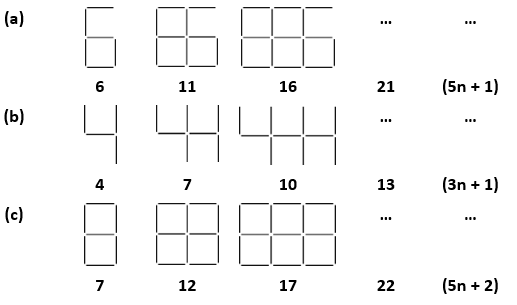If the number of digits formed is taken to be n, the number of segments required to form n digits is given by the algebraic expression appearing on the right of each pattern.

How many segments are required to form 5, 10, 100 digits of the kind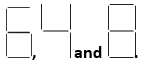Solution:

Segments required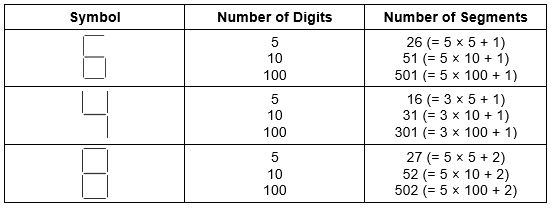2. Use the given algebraic expression to complete the table of number patterns.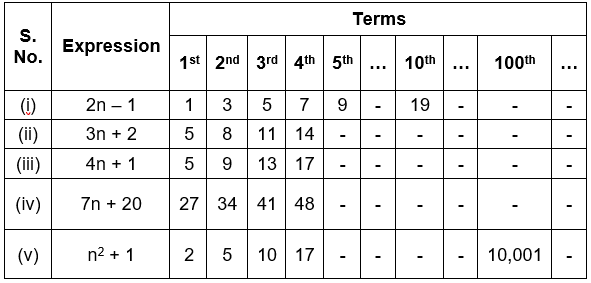Solution:

Evaluating the value of expressions with respective values from the columns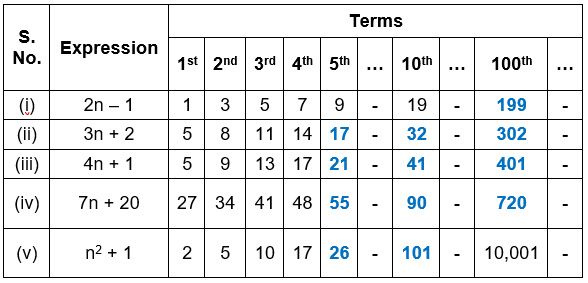With this we come to the end of NCERT Solutions for Class 7 Maths Chapter 12 Algebraic Expressions. We hope these helped you study your subject.

Download NCERT Solutions for Class 7 Maths Chapter 12 – Algebraic Expressions